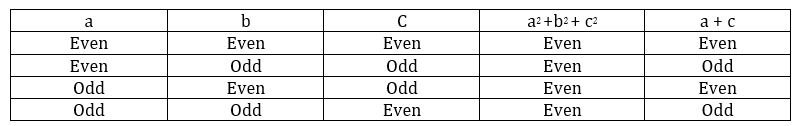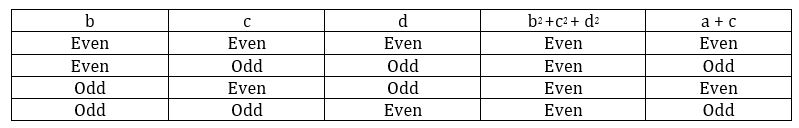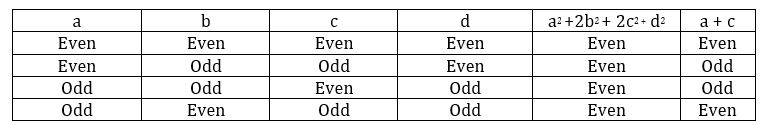GMAT Question of the Day - Daily to your Mailbox; hard ones only

 It is currently 07 Dec 2019, 01:30### GMAT Club Daily Prep

#### Thank you for using the timer - this advanced tool can estimate your performance and suggest more practice questions. We have subscribed you to Daily Prep Questions via email.

Customized
for You

we will pick new questions that match your level based on your Timer History

Track

every week, we’ll send you an estimated GMAT score based on your performance

Practice
Pays

we will pick new questions that match your level based on your Timer History

#### Not interested in getting valuable practice questions and articles delivered to your email? No problem, unsubscribe here.# If a, b, c, d are 4 non-negative integers. Is (a+c) even?

Author Message
TAGS:

### Hide Tags

e-GMAT RepresentativeV
Joined: 04 Jan 2015
Posts: 3158
If a, b, c, d are 4 non-negative integers. Is (a+c) even?  [#permalink]

### Show Tags00:00

Difficulty:35% (medium)

Question Stats:72% (02:09) correct28% (02:06) wrongbased on 201 sessions

### HideShow timer Statistics

e-GMAT Question:

If a, b, c, d are 4 non-negative integers. Is (a+c) even?

1. $$a^2 +b^2 + c^2$$ is even
2. $$b^2 + c^2 + d^2$$ is even

A) Statement (1) ALONE is sufficient, but statement (2) alone is not sufficient.
B) Statement (2) ALONE is sufficient, but statement (1) alone is not sufficient.
C) BOTH statements TOGETHER are sufficient, but NEITHER statement ALONE is sufficient.
D) EACH statement ALONE is sufficient.
E) Statements (1) and (2) TOGETHER are NOT sufficient

This is

Question 4 of The e-GMAT Number Properties Marathon

Go to

Question 5 of the Marathon

_________________

Originally posted by EgmatQuantExpert on 27 Feb 2018, 10:20.
Last edited by EgmatQuantExpert on 13 Aug 2018, 02:46, edited 2 times in total.
Current StudentP
Joined: 07 Jan 2016
Posts: 1081
Location: India
GMAT 1: 710 Q49 V36Re: If a, b, c, d are 4 non-negative integers. Is (a+c) even?  [#permalink]

### Show Tags

1
EgmatQuantExpert wrote:

Question:

If a, b, c, d are 4 non-negative integers. Is (a+c) even?

1. $$a^2 +b^2 + c^2$$ is even
2. $$b^2 + c^2 + d^2$$ is even

A) Statement (1) ALONE is sufficient, but statement (2) alone is not sufficient.
B) Statement (2) ALONE is sufficient, but statement (1) alone is not sufficient.
C) BOTH statements TOGETHER are sufficient, but NEITHER statement ALONE is sufficient.
D) EACH statement ALONE is sufficient.
E) Statements (1) and (2) TOGETHER are NOT sufficient

we know

even + even = even
odd + odd = even

so

1) $$a^2 +b^2 + c^2$$ is even now here a b c are even or 2 out a/b/c are odd and one is even
now a and b even c odd
a+c not even
now a and c odd b even
a+c even
insufficient

2) $$b^2 + c^2 + d^2$$ is even
insufficient similar to
b c even d odd
we don't know value of a

now
combining
$$a^2 +b^2 + c^2$$ + $$b^2 + c^2 + d^2$$ = $$a^2 + 2 b^2 + 2 c^2 + d^2$$
here a^2 + d^2 is even as b/c can be odd or even and still the result is even

we know nothing about C being even/odd

(E) imo
Senior PS ModeratorV
Joined: 26 Feb 2016
Posts: 3300
Location: India
GPA: 3.12
Re: If a, b, c, d are 4 non-negative integers. Is (a+c) even?  [#permalink]

### Show Tags

We need to know the following odd-even properties
even^2 = even | odd^2 = odd | even + even = even | odd + odd = even | even + odd = odd

Is a+c even?

1. $$a^2 + b^2 + c^2$$ is even
If $$a^2$$, $$b^2$$,and $$c^2$$ are all even, then a+c is even
If $$a^2$$ and $$b^2$$ are odd and $$c^2$$ is even, a+c is odd (Insufficient)

2. $$b^2 + c^2 + d^2$$ is even
This statement contains no information about a.
We cannot say if a+c is even or not. (Insufficient)

On combining the information in both the statements,
We can have a case where a,b, and d are odd, whereas c is even. Here, a+c is odd
But, if a,b,c, and d are all even, then a+c will be even (Insufficient - Option E)
_________________
You've got what it takes, but it will take everything you've got
e-GMAT RepresentativeV
Joined: 04 Jan 2015
Posts: 3158
Re: If a, b, c, d are 4 non-negative integers. Is (a+c) even?  [#permalink]

### Show Tags

Solution:

Step 1: Analyse Statement 1:
$$a^2 +b^2 + c^2$$ is Even
• If $$a^2 +b^2 + c^2$$ is Even, it gives rise to two possibilities:
o Either one of $$a/b/c$$ is Even and the rest two are Odd
o Or, all the three numbers: $$a, b$$ and $$c$$are Even.
Per our conceptual knowledge, let’s make a table to understand this.It is clear from the table that the even-odd nature of $$(a + c)$$varies, and we cannot conclude anything about it.
As we do not know the exact even-odd nature of$$(a + c)$$,
Statement 1 alone is NOT sufficient to answer the question.
Hence, we can eliminate answer choices A and D.
Step 2: Analyse Statement 2:
$$b^2 +c^2 + d^2$$ is Even
• If $$b^2 +c^2 + d^2$$ is Even, it gives rise to two possibilities:
o Either one of $$b/c/d$$ is Even and rest two are Odd
o Or, all the three numbers: $$b, c$$ and $$d$$ are Even.
Let’s make a table to understand this.It is clear from the table that the even-odd nature of $$(a + c)$$ varies, and we cannot conclude anything about it.
As we do not know the exact even-odd nature of $$(a + c)$$,
Statement 2 alone is NOT sufficient to answer the question.
Hence, we can eliminate answer choice B.
Step 3: Combine both Statements:
From the first statement, we have: $$a^2 +b^2 + c^2$$ = Even
From the second statement, we have: $$b^2 +c^2 + d^2$$ = Even
Combining both the statements, we have: a$$^2 +b^2 + c^2 + b^2 +c^2 + d^2$$ = Even + Even = Even
Therefore, $$a^2 +2b^2 + 2c^2 + d^2$$ is even
We know that, $$2b^2 + 2c^2$$ is always even irrespective of the even-odd nature of $$b$$ and $$c$$, since they are multiplied by an even number $$(2)$$
So, our expression reduces to: $$a^2 + d^2$$ is even. This will lead to two possibilities:
• Either both a and d are Odd.
• Or both a and d are Even.
Per our conceptual understanding, let us draw a table combining both the tables from Step 3 and Step 4 to see how will the even odd nature of the numbers affect the even-odd nature of the expression: $$(a + c)$$:From the table it is evident that the even-odd nature of the expression $$(a + c)$$ cannot be determined uniquely.
By combining both statements we did not get a unique answer.
_________________
Non-Human UserJoined: 09 Sep 2013
Posts: 13721
Re: If a, b, c, d are 4 non-negative integers. Is (a+c) even?  [#permalink]

### Show Tags

Hello from the GMAT Club BumpBot!

Thanks to another GMAT Club member, I have just discovered this valuable topic, yet it had no discussion for over a year. I am now bumping it up - doing my job. I think you may find it valuable (esp those replies with Kudos).

Want to see all other topics I dig out? Follow me (click follow button on profile). You will receive a summary of all topics I bump in your profile area as well as via email.
_________________Re: If a, b, c, d are 4 non-negative integers. Is (a+c) even?   [#permalink] 22 May 2019, 12:21
Display posts from previous: Sort by

# If a, b, c, d are 4 non-negative integers. Is (a+c) even?